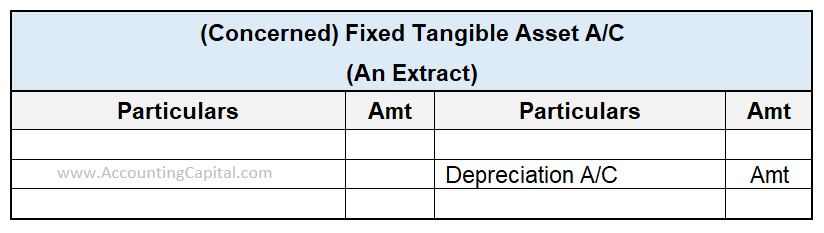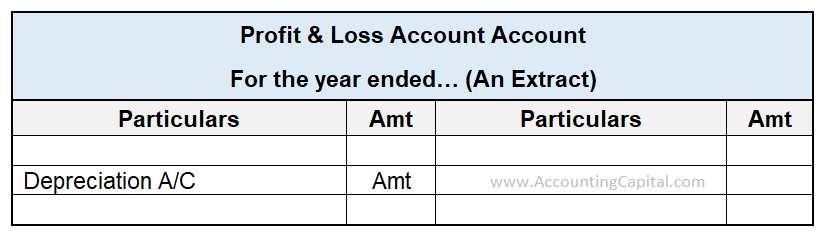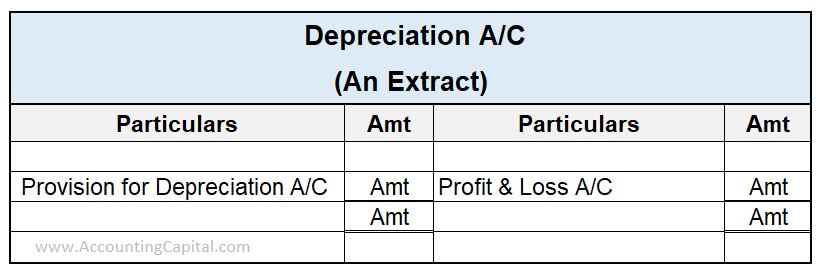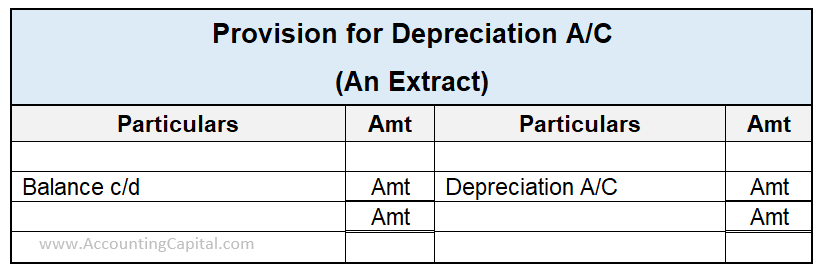# Difference Between Depreciation and Provision for Depreciation

## Depreciation Vs Provision for Depreciation

Depreciation is an accounting process of distributing the cost of an asset over its estimated useful life as per the matching concept. However, when such depreciation is accumulated in a separate account till the date of the concerned asset’s disposal or sale, it is called provision for depreciation.

In other words, provision for depreciation refers to the amount of depreciation accumulated over the useful life of an asset and is also known as accumulated depreciation.

Thus, we can say that the two terms are quite inter-linked but are not the same. Following are the key differences between the two;

 Depreciation Provision for Depreciation 1) Gradual fall in the value of fixed tangible assets due to normal wear & tear or obsolescence over their expected useful life is called depreciation. 1) Provision for depreciation is a provision created against the depreciation accumulated on a fixed tangible asset over its expected useful life. 2) It is a nominal account. 2) It is a real account. 3) Being an expense, depreciation is reflected in the profit & loss account and not in the balance sheet. 3)Provision for depreciation is reflected in the balance sheet as it is a contra asset account. 4) The asset account is recorded in the books at written down value. It is the value that remains after charging depreciation for that year. 4) The asset is recorded in the books at the original cost. The balance of the “provision for depreciation account” is shown on the liability side of a balance sheet. 5) Depreciation is an expense. Hence, its balance is transferred to the profit and loss account. 5) Provision for depreciation is a contra asset. Thus, it will always have a credit balance.

Related Topic – Provision for depreciation in the trial balance

The most commonly used methods for charging depreciation are;

a) Straight Line Value Method – In this method, the depreciation is always charged on the original cost of the tangible asset. Thus, the amount of depreciation charged remains constant each year irrespective of asset usage. Hence, it is also called the fixed installment method of charging depreciation.

b) Written Down Value Method – In this method, the depreciation in the first year is charged on the historical cost of the asset but in the following years, it is charged on the respective asset’s written down value. Therefore, this method is also called the diminishing balance method.

Related Topic – Which contra account is used in recording depreciation?

## Depreciation

Depreciation refers to the fall in the value of fixed tangible assets over its expected useful life. Various reasons behind the depreciation of fixed tangible assets can be wear & tear, obsolescence, consumption, and so on.

Example

Suppose Berkshire Hathaway Inc. depreciates its equipment having a useful life of 10 years, at @10% p.a. using the diminishing balance method. The opening balance of the equipment is 90,000.

Thus, the depreciation in the equipment account in the books of Berkshire Hathaway Inc. for a period of say 3 years will be as follows;

 Equipment A/C Particular Amount Particular Amount Balance b/d 90,000 Depreciation 9,000 Balance c/d 81,000 Total 90,000 Total 90,000 Balance b/d 81,000 Depreciation 8,100 Balance c/d 72,900 Total 81,000 Total 81,000
 Balance b/d 72,900 Depreciation 7,290 Balance c/d 65,610 Total 72,900 Total 72,900

Alternatively, if in the same example the depreciation was charged @10% p.a. using the Straight Line method or Fixed Installment Method, an amount of 9,000(90,000*10%) would be credited each year and the balance of the equipment account at the end of the third year would be 90,000 – 9,000*3 i.e. 63,000.

Related Topic – Is depreciation an operating expense?

Treatment of Depreciation in the Books

Firstly, credit the amount of depreciation to the respective tangible asset account because it will reduce the value of the asset.Now, debit the depreciation account to the profit & loss account as depreciation is an indirect expense for the business.Related Topic – Can depreciation be charged in the year of sale?

## Provision for Depreciation

Provision for Depreciation refers to the total amount of depreciation charged on a fixed tangible asset till the date of its sale or disposal. Hence, it is a contra asset and is also called accumulated depreciation.

Example

Let’s assume that Toyota Group has an opening balance of machinery of 70,000 & it charges depreciation on its machinery at @5% p.a. using the straight-line method. Further, the firm maintains a provision for depreciation account for the same.

Therefore, the provision for depreciation account in the books of the Toyota Group for a period supposing 5 years will be as follows;

Depreciation = 5% * 70,000 = 3500 annually.

 Provision for Depreciation A/C Particular Amount Particular Amount Balance c/d 3,500 Depreciation 3,500 Total 3,500 Total 3,500 Balance b/d 3,500 Balance c/d 7,000 Depreciation 3,500 Total 7,000 Total 7,000
 Balance b/d 7,000 Balance c/d 10,500 Depreciation 3,500 Total 10,500 Total 10,500
 Balance b/d 10,500 Balance c/d 14,000 Depreciation 3,500 Total 14,000 Total 14,000
 Balance b/d 14,000 Balance c/d 17,500 Depreciation 3,500 Total 17,500 Total 17,500

Related Topic – Can assets have a credit balance?

Treatment of Provision for Depreciation in the Books

Depreciation is an indirect expense for an enterprise. Hence, debit the firm’s profit & loss account by the depreciation amount.

Now, transfer the amount of depreciation charged during a given financial year to the credit side of the provision for depreciation account as it will increase the amount of accumulated depreciation.

The accumulated depreciation in the provision account shall be transferred to the asset account at the time of its sale or disposal.>Related Long Quiz for Practice Quiz 39 – Depreciation

###### Subscribe
* indicates required